SSC CGL Previous Year Questions: Simplification and Square & Cube Root- 3

# SSC CGL Previous Year Questions: Simplification and Square & Cube Root- 3 - SSC CGL

Test Description

## 30 Questions MCQ Test SSC CGL (Tier - 1) - Previous Year Papers (Topic Wise) - SSC CGL Previous Year Questions: Simplification and Square & Cube Root- 3

SSC CGL Previous Year Questions: Simplification and Square & Cube Root- 3 for SSC CGL 2023 is part of SSC CGL (Tier - 1) - Previous Year Papers (Topic Wise) preparation. The SSC CGL Previous Year Questions: Simplification and Square & Cube Root- 3 questions and answers have been prepared according to the SSC CGL exam syllabus.The SSC CGL Previous Year Questions: Simplification and Square & Cube Root- 3 MCQs are made for SSC CGL 2023 Exam. Find important definitions, questions, notes, meanings, examples, exercises, MCQs and online tests for SSC CGL Previous Year Questions: Simplification and Square & Cube Root- 3 below.
Solutions of SSC CGL Previous Year Questions: Simplification and Square & Cube Root- 3 questions in English are available as part of our SSC CGL (Tier - 1) - Previous Year Papers (Topic Wise) for SSC CGL & SSC CGL Previous Year Questions: Simplification and Square & Cube Root- 3 solutions in Hindi for SSC CGL (Tier - 1) - Previous Year Papers (Topic Wise) course. Download more important topics, notes, lectures and mock test series for SSC CGL Exam by signing up for free. Attempt SSC CGL Previous Year Questions: Simplification and Square & Cube Root- 3 | 30 questions in 30 minutes | Mock test for SSC CGL preparation | Free important questions MCQ to study SSC CGL (Tier - 1) - Previous Year Papers (Topic Wise) for SSC CGL Exam | Download free PDF with solutions
 1 Crore+ students have signed up on EduRev. Have you?
SSC CGL Previous Year Questions: Simplification and Square & Cube Root- 3 - Question 1

### Which is greater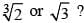(SSC CHSL 2013)

Detailed Solution for SSC CGL Previous Year Questions: Simplification and Square & Cube Root- 3 - Question 1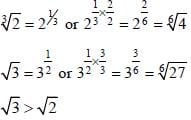SSC CGL Previous Year Questions: Simplification and Square & Cube Root- 3 - Question 2

### The value of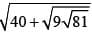is       (SSC CHSL 2013)

Detailed Solution for SSC CGL Previous Year Questions: Simplification and Square & Cube Root- 3 - Question 2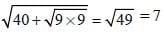SSC CGL Previous Year Questions: Simplification and Square & Cube Root- 3 - Question 3

### The greatest among the following numbers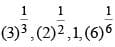is:       (SSC Sub. Ins. 2013)

Detailed Solution for SSC CGL Previous Year Questions: Simplification and Square & Cube Root- 3 - Question 3

LCM of 3, 2 and 6 = 6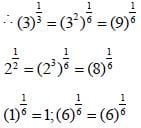SSC CGL Previous Year Questions: Simplification and Square & Cube Root- 3 - Question 4

The value ofis:      (SSC Sub. Ins. 2013)

Detailed Solution for SSC CGL Previous Year Questions: Simplification and Square & Cube Root- 3 - Question 4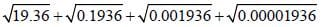= 4.4 + 0.44 + 0.044 + 0.0044 = 4.8884

SSC CGL Previous Year Questions: Simplification and Square & Cube Root- 3 - Question 5

The value of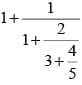is:         (SSC Sub. Ins. 2013)

Detailed Solution for SSC CGL Previous Year Questions: Simplification and Square & Cube Root- 3 - Question 5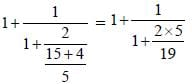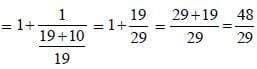SSC CGL Previous Year Questions: Simplification and Square & Cube Root- 3 - Question 6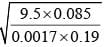equals        (SSC Multitasking 2013)

Detailed Solution for SSC CGL Previous Year Questions: Simplification and Square & Cube Root- 3 - Question 6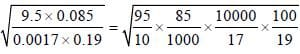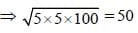SSC CGL Previous Year Questions: Simplification and Square & Cube Root- 3 - Question 7

The simplified value of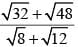is        (SSC Multitasking 2013)

Detailed Solution for SSC CGL Previous Year Questions: Simplification and Square & Cube Root- 3 - Question 7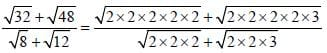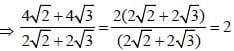SSC CGL Previous Year Questions: Simplification and Square & Cube Root- 3 - Question 8

If 21 is added to a number, it becomes 7 less than thrice of the number. Then the number is       (SSC CGL 2012)

Detailed Solution for SSC CGL Previous Year Questions: Simplification and Square & Cube Root- 3 - Question 8

If the number be x, then
x + 21 = 3x – 7
⇒ 3x – x = 21 + 7
⇒ 2x = 28
⇒ x = 14

SSC CGL Previous Year Questions: Simplification and Square & Cube Root- 3 - Question 9

The simplest value of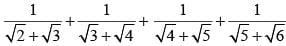is       (SSC CGL 2012)

Detailed Solution for SSC CGL Previous Year Questions: Simplification and Square & Cube Root- 3 - Question 9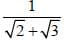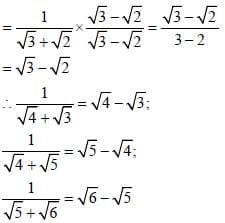∴ Expression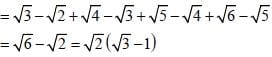SSC CGL Previous Year Questions: Simplification and Square & Cube Root- 3 - Question 10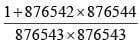is equal to       (SSC CGL 2012)

Detailed Solution for SSC CGL Previous Year Questions: Simplification and Square & Cube Root- 3 - Question 10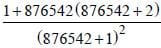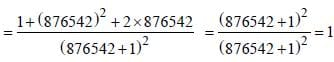SSC CGL Previous Year Questions: Simplification and Square & Cube Root- 3 - Question 11

If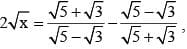then the value of x is:       (SSC CGL 2nd Sit. 2012)

Detailed Solution for SSC CGL Previous Year Questions: Simplification and Square & Cube Root- 3 - Question 11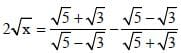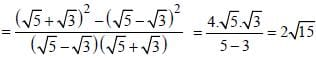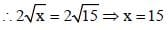SSC CGL Previous Year Questions: Simplification and Square & Cube Root- 3 - Question 12

By what least number should 675 be multiplied to obtain a number which is a perfect cube?        (SSC CGL 2012)

Detailed Solution for SSC CGL Previous Year Questions: Simplification and Square & Cube Root- 3 - Question 12

675 = 5 × 5 × 3 × 3 × 3
= 33 × 52
∴ Required number = 5

SSC CGL Previous Year Questions: Simplification and Square & Cube Root- 3 - Question 13

A farmer divides his herd of n cows among his four sons, so that the first son gets one–half the herd, the second one– fourth, the third son 1/5 and the fourth son 7 cows. Then thevalue of n is       (SSC CGL 2012)

Detailed Solution for SSC CGL Previous Year Questions: Simplification and Square & Cube Root- 3 - Question 13

According to the question,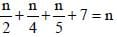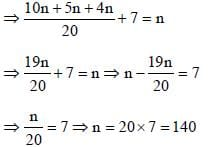SSC CGL Previous Year Questions: Simplification and Square & Cube Root- 3 - Question 14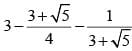is equal to        (SSC CGL 2012)

Detailed Solution for SSC CGL Previous Year Questions: Simplification and Square & Cube Root- 3 - Question 14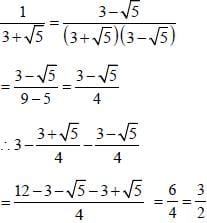SSC CGL Previous Year Questions: Simplification and Square & Cube Root- 3 - Question 15

If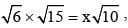then the value of x is        (SSC CGL 2012)

Detailed Solution for SSC CGL Previous Year Questions: Simplification and Square & Cube Root- 3 - Question 15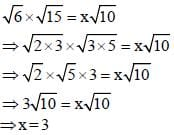SSC CGL Previous Year Questions: Simplification and Square & Cube Root- 3 - Question 16

The value of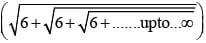is equal to       (SSC CGL 1st Sit. 2012)

Detailed Solution for SSC CGL Previous Year Questions: Simplification and Square & Cube Root- 3 - Question 16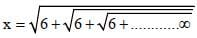On squaring,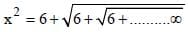⇒ x2 = 6 + x
⇒ x2 – x – 6 = 0
⇒ x2 – 3x + 2x – 6 = 0
⇒ x (x – 3) + 2 (x –3) = 0
⇒ (x – 3) (x + 2) = 0
⇒ x = 3 because x ≠ -2
By trick 3 x 2 = 6

SSC CGL Previous Year Questions: Simplification and Square & Cube Root- 3 - Question 17

From 9.00 AM to 2.00 PM, the temperature rose at a constant rate from 21ºC to 36ºC. What was the temperature at noon ?       (SSC CHSL 2012)

Detailed Solution for SSC CGL Previous Year Questions: Simplification and Square & Cube Root- 3 - Question 17

Time difference between 9.00 A.M & 2.00 P.M = 5 hours
Temperature difference between 21°C & 36°C = 36 – 21 = 15°C
Now, Time difference between 9.00 A.M & 12.00 Noon = 3 hrs.
In 5 hours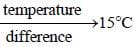So, In 3 hours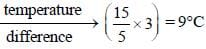So, temperature at noon = 21 + 9 = 30°C

SSC CGL Previous Year Questions: Simplification and Square & Cube Root- 3 - Question 18

If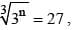then the value of n is:       (SSC CHSL 2012)

Detailed Solution for SSC CGL Previous Year Questions: Simplification and Square & Cube Root- 3 - Question 18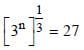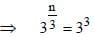Comparing, n/3 = 3
x = 9

SSC CGL Previous Year Questions: Simplification and Square & Cube Root- 3 - Question 19

If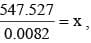then the value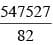is :      (SSC CHSL 2012)

Detailed Solution for SSC CGL Previous Year Questions: Simplification and Square & Cube Root- 3 - Question 19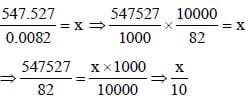SSC CGL Previous Year Questions: Simplification and Square & Cube Root- 3 - Question 20

The value of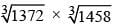is       (SSC Sub. Ins. 2012)

Detailed Solution for SSC CGL Previous Year Questions: Simplification and Square & Cube Root- 3 - Question 20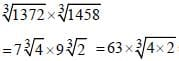= 63 x 2 = 126

SSC CGL Previous Year Questions: Simplification and Square & Cube Root- 3 - Question 21

What is the value of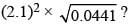(SSC Sub. Ins. 2012)

Detailed Solution for SSC CGL Previous Year Questions: Simplification and Square & Cube Root- 3 - Question 21

Expression is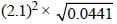= 4.41 × 0.21 = 0.9261

SSC CGL Previous Year Questions: Simplification and Square & Cube Root- 3 - Question 22

Last year my age was a perfect square number. Next year it will be a cubic number. What is my present age?       (SSC Sub. Ins. 2012)

Detailed Solution for SSC CGL Previous Year Questions: Simplification and Square & Cube Root- 3 - Question 22

By going options, 26 years is the present age. Present age be 26, then last year age was 25 which represents a perfect square and next year age would be 27 which represents a cubic number.

SSC CGL Previous Year Questions: Simplification and Square & Cube Root- 3 - Question 23

A student was asked to divide a number by 6 and add 12 to the quotient. He, however, first added 12 to the number and then divided it by 6, getting 112 as the answer. The correct answer should have been       (SSC CGL 2nd Sit. 2011)

Detailed Solution for SSC CGL Previous Year Questions: Simplification and Square & Cube Root- 3 - Question 23

Let the number be x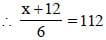⇒ x + 12 = 672
⇒ x = 672 – 12 = 660
∴ Correct answer = (660/6) + 12 = 110 + 12 = 122

SSC CGL Previous Year Questions: Simplification and Square & Cube Root- 3 - Question 24

The value of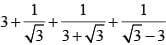is       (SSC CGL 2nd Sit. 2011)

Detailed Solution for SSC CGL Previous Year Questions: Simplification and Square & Cube Root- 3 - Question 24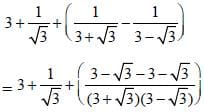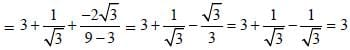SSC CGL Previous Year Questions: Simplification and Square & Cube Root- 3 - Question 25

If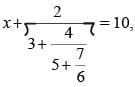then the value of x is        (SSC CGL 2nd Sit. 2011)

Detailed Solution for SSC CGL Previous Year Questions: Simplification and Square & Cube Root- 3 - Question 25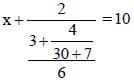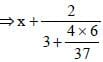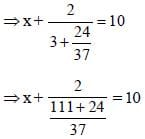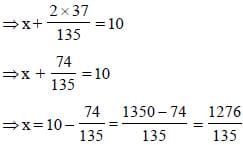SSC CGL Previous Year Questions: Simplification and Square & Cube Root- 3 - Question 26

If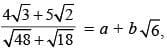then the values of a and b arerespectively       (SSC CGL 2nd Sit. 2011)

Detailed Solution for SSC CGL Previous Year Questions: Simplification and Square & Cube Root- 3 - Question 26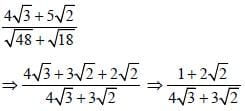By Rationalising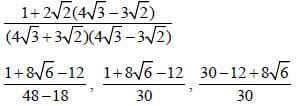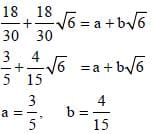SSC CGL Previous Year Questions: Simplification and Square & Cube Root- 3 - Question 27

Find a number, one–seventh of which exceeds its eleventh part by 100.        (SSC CGL 2nd Sit. 2011)

Detailed Solution for SSC CGL Previous Year Questions: Simplification and Square & Cube Root- 3 - Question 27

Let the number be x.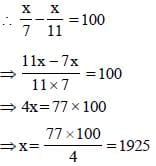SSC CGL Previous Year Questions: Simplification and Square & Cube Root- 3 - Question 28

If the sum of two numbers be multiplied by each number separately, the products so obtained are 247 and 114. The sum of the numbers is        (SSC CGL 2nd Sit. 2011)

Detailed Solution for SSC CGL Previous Year Questions: Simplification and Square & Cube Root- 3 - Question 28

Let the numbers be x and y.
∴ x (x + y) = 247
and y (x + y) = 114
⇒ x2 + xy = 247 and xy + y2 = 114
x2 + xy + xy + y2 = 247 + 114
⇒ x2 + 2xy + y2 = 361
⇒ (x + y)2 = 192 ⇒  x + y = 19

SSC CGL Previous Year Questions: Simplification and Square & Cube Root- 3 - Question 29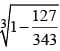is equal to       (SSC CGL 2nd Sit. 2011)

Detailed Solution for SSC CGL Previous Year Questions: Simplification and Square & Cube Root- 3 - Question 29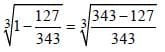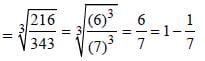SSC CGL Previous Year Questions: Simplification and Square & Cube Root- 3 - Question 30

If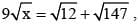then x = ?       (SSC CGL 2011)

Detailed Solution for SSC CGL Previous Year Questions: Simplification and Square & Cube Root- 3 - Question 30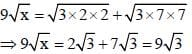∴ x = 3

## SSC CGL (Tier - 1) - Previous Year Papers (Topic Wise)

250 tests
Information about SSC CGL Previous Year Questions: Simplification and Square & Cube Root- 3 Page
In this test you can find the Exam questions for SSC CGL Previous Year Questions: Simplification and Square & Cube Root- 3 solved & explained in the simplest way possible. Besides giving Questions and answers for SSC CGL Previous Year Questions: Simplification and Square & Cube Root- 3, EduRev gives you an ample number of Online tests for practice

250 tests